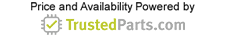Information Technology

# Creating Neural Networks in Python

16 June 2017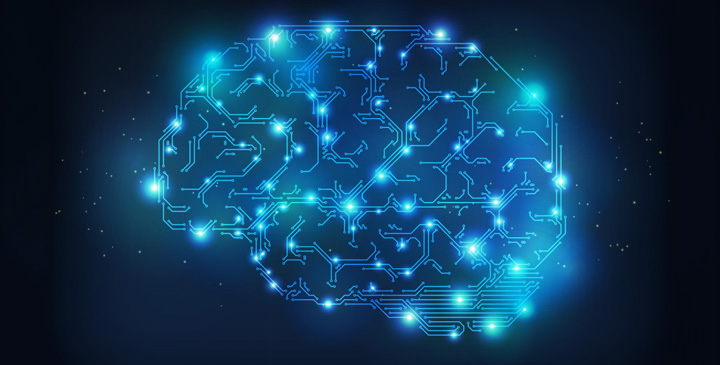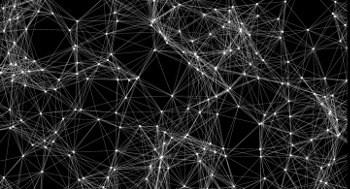An illustrative example of an artificial neural network showing nodes and the links between them. Image credit: Jonathan HeathcoteArtificial neural networks are machine learning frameworks that simulate the biological functions of natural brains to solve complex problems like image and speech recognition with a computer. In real brains, information is processed by building block cells called neurons. A detailed understanding of exactly how this occurs is the subject of ongoing research. But at a basic level, neurons receive signals through their dendrites and output signals to other neurons through axons over synapses.

Neural networks attempt to mimic the behavior of real neurons by modeling the transmission of information between nodes (simulated neurons) of an artificial neural network. The networks “learn” in an adaptive way, continuously adjusting parameters until the correct output is produced for a given input. Signal intensities along node connections are adjusted through activation functions by evaluating prediction errors in a trial and error process until the network learns the best answer.

Library Packages

Packages for coding neural networks exist in most popular programming languages, including Matlab, Octave, C, C++, C#, Ruby, Perl, Java, Javascript, PHP and Python. Python is a high-level programming language designed for code readability and efficient syntax that allows expression of concepts in fewer lines of code than languages like C++ or Java. Two Python libraries that have particular relevance to creating neural networks are NumPy and Theano.

NumPy is a Python package that contains a variety of tools for scientific computing, including an N-dimensional array object, broadcasting functions, and linear algebra and random number capabilities. NumPy offers an efficient multi-dimensional container of generic data with arbitrary definition of data types, allowing seamless integration with a wide variety of databases. NumPy’s functions serve as fundamental building blocks for scientific computing, and many of its features are integral in developing neural networks in Python.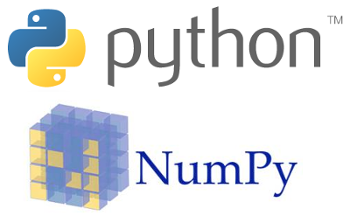NumPy is a library package for the Python programming language that can be used to develop neural networks, among other scientific computing tasks.

Theano—a Python library that defines, optimizes and evaluates mathematical expressions—integrates neatly with NumPy. It has unit-testing and self-verification features to identify errors; efficient symbolic differentiation; dynamic generation of C code for quick expression evaluation; and is capable of utilizing the graphics processing unit (GPU) transparently for very fast data-intensive computations. It can model computations as graphs and use the chain rule to calculate complex gradients. This is particularly applicable to neural networks because they are readily expressed as graphs of computations. Utilizing the Theano library, neural networks can be written more concisely and execute much faster, especially if a GPU is employed.

Other Python libraries designed to facilitate machine learning include:

· scikit-learn: a machine learning library for Python built on NumPy, SciPy and matplotlib.

· TensorFlow: a machine intelligence library that uses data flow graphs to model neural networks, capable of distributed computing across multiple CPUs or GPUs.

· Keras: a high level neural network application program interface (API) able to run on top of TensorFlow or Theano.

· Lasagne: a lightweight library for constructing neural networks in Theano. Lasagne serves as a middle ground between the high level abstractions of Keras and the low-level programming of Theano.

· mxnet: a deep learning framework capable of distributed computing with a large selection of language bindings, including C++, R, Scala and Julia.

Three Layer Neural Network

A simple three layer neural network can be programmed in Python as seen in the accompanying image from iamtrask’s neural network python tutorial. This basic network’s only external library is NumPy (assigned to ‘np’). It has an input layer (represented as X), a hidden layer (l1) and an output layer (l2). The input layer is specified by the input data, which the network attempts to correlate with the output layer across the second hidden layer by approximating the correct answer in a trial and error process as the network is trained.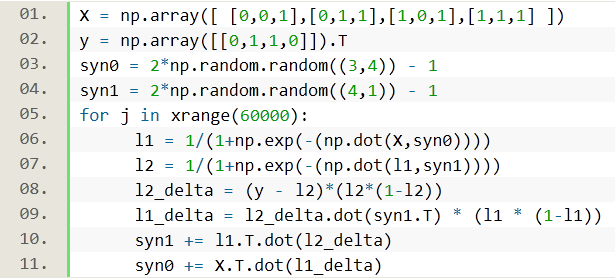A three layer neural network in Python. Image credit: iamtrask (Click to enlarge)The first two lines of this Python neural network assign values to the input (X) and output (Y) datasets. The next two lines initialize the random weights (syn0 and syn1), which are analogous to synapses connecting the network’s layers and are used to predict the output given the input data. Line 5 sets up the main loop of the neural network that iterates many times (60,000 iterations in this case) to train the network for the correct solution. Lines 6 and 7 create a predicted answer using a sigmoid activation function and feed it forward through layers l1 and l2. Lines 8 and 9 assess the errors in layers l2 and l1. Lines 10 and 11 update the weights syn1 and syn0 with the error information from lines 8 and 9. The process happening in lines 8 through 11 is referred to as backpropagation, which uses the chain rule for derivative calculation. In this approach, each of the network’s weights is updated to be closer to the real output, minimizing the error of each “synapse” as the iterative procedure progresses.

Additional operations that can improve this basic neural network for certain datasets include adding a bias and normalizing the input data. A bias allows shifting the activation function to the left or right to better fit the data. Normalizing the input data, meanwhile, is important for obtaining accurate results for some datasets, as many neural network implementations are sensitive to feature scaling. Examples of data normalization include scaling each input value to fall between 0 and 1, or standardizing the inputs to have a mean of 0 and a variance of 1.

With the capability to adaptively learn, neural networks have a powerful advantage over conventional programming techniques. In particular, they are useful in applications that are difficult for traditional code to handle like image recognition, speech synthesis, decision making and forecasting. As the processing power of computer hardware continues to increase and new architectures are developed, neural networks promise to be influential tools able to handle increasingly complex tasks that previously only humans could manage.